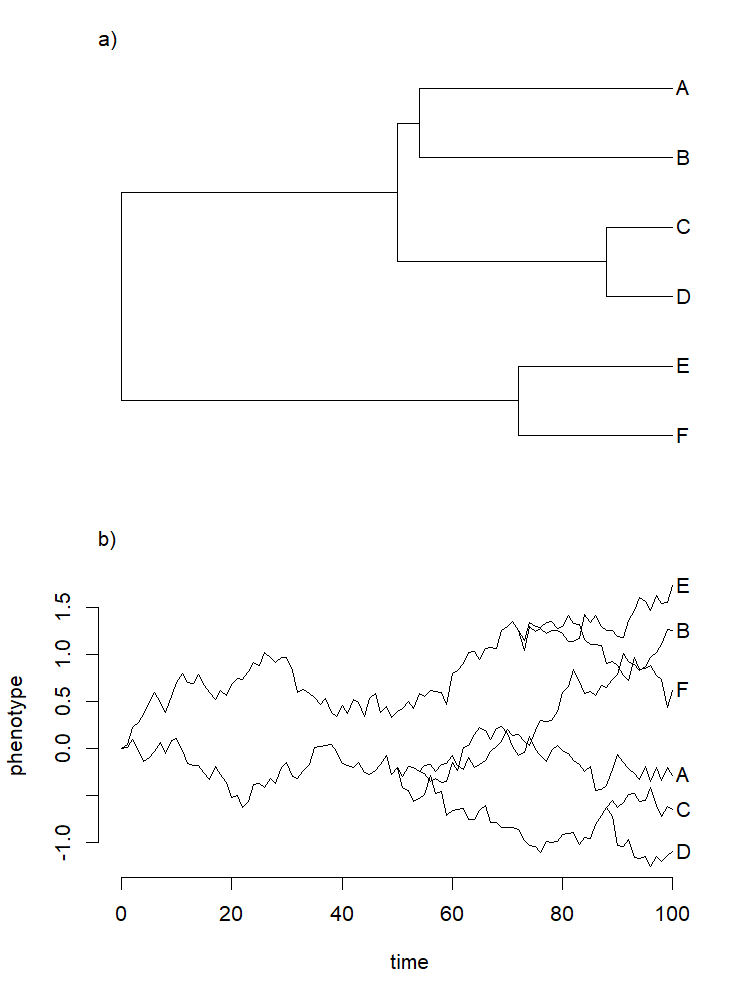## Thursday, November 28, 2019

### New function for graphical simulation of Brownian motion on a tree

Here's a new function that creates a two panel plot. The first panel contains a simulated, discrete-time, pure-birth phylogeny of N taxa. The second panel gives the results of a discrete-time Brownian (random walk) simulation on the tree. I developed it for use in teaching, but will add it to phytools shortly:

``````simBMphylo<-function(n,t,sig2,plot=TRUE,...){
b<-exp((log(n)-log(2))/t)-1
tree<-pbtree(b=b,d=0,t=t,n=n,type="discrete",
tip.label=if(n<=26) LETTERS[n:1] else NULL,
quiet=TRUE)
H<-nodeHeights(tree)
root<-Ntip(tree)+1
xx<-list()
for(i in 1:nrow(tree\$edge)){
x<-rnorm(n=tree\$edge.length[i],sd=sqrt(sig2))
x<-c(0,cumsum(x))
if(tree\$edge[i,1]!=root){
ii<-which(tree\$edge[,2]==tree\$edge[i,1])
x<-x+xx[[ii]][length(xx[[ii]])]
}
xx[[i]]<-x
}
object<-list(tree=tree,x=xx)
class(object)<-"simBMphylo"
if(plot) plot(object,...)
invisible(object)
}

plot.simBMphylo<-function(x,...){
xx<-x\$x
tree<-x\$tree
H<-nodeHeights(tree)
par(mfrow=c(2,1))
plotTree(tree,mar=c(2.1,4.1,3.1,1.1),
xlim=c(0,1.05*max(H)),lwd=1)
plot.new()
par(mar=c(5.1,4.1,3.1,1.1))
plot.window(xlim=c(0,1.05*max(H)),ylim=range(xx))
axis(1)
axis(2)
for(i in 1:length(xx))
lines(H[i,1]:H[i,2],xx[[i]])
for(i in 1:Ntip(tree)){
ii<-which(tree\$edge[,2]==i)
text(max(H),xx[[ii]][length(xx[[ii]])],
tree\$tip.label[i],pos=4,offset=0.4/3)
}
title(xlab="time",ylab="phenotype")
}

print.simBMphylo<-function(x,...){
cat(paste("\nObject of class \"simBMphylo\" with",Ntip(x\$tree),"taxa.\n"),
sep="")
cat("To print use plot method.\n\n")
}
``````

Let's see how it works:

``````library(phytools)
set.seed(30)
simBMphylo(n=6,t=100,sig2=0.01)
``````That's it. Happy thanksgiving.